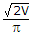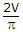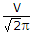# Electronics and Communication Engineering - Power Electronics

### Exercise :: Power Electronics - Section 7

16.

Assertion (A): A GTO can be turned on and turned off by gate pulses

Reason (R): A GTO can be turned off by negative gate pulse.

 A. Both A and R are correct and R is correct explanation of A B. Both A and R correct but R is not correct explanation of A C. A is correct but R is wrong D. A is wrong but R is correct

Explanation:

No answer description available for this question. Let us discuss.

17.

In a half bridge inverter circuit fed by a battery of voltage V, the rms value of fundamental component of output voltage is

 A. 2 V B.C.D.Explanation:

No answer description available for this question. Let us discuss.

18.

In a full bridge single phase inverter feeding RLC load

 A. one thyristor and one diode conduct simultaneously B. two thyristors conduct simultaneously C. two thyristors or two diodes conduct simultaneously D. four thyristors conduct simultaneously

Explanation:

No answer description available for this question. Let us discuss.

19.

It is possible to use a freewheeling diode in

 A. single phase half wave controlled rectifier as well as in single phase full wave controlled rectifier (M - 2 connection) B. single phase half wave controlled rectifier but not in single phase full wave controlled rectifier (M - 2 connection) C. single phase full wave controlled rectifier (M - 2 connection) but not in single phase half wave controlled rectifier D. all controlled rectifier circuits

Explanation:

No answer description available for this question. Let us discuss.

20.

Assertion (A): When the anode current of an SCR starts increasing during turn on process, the anode cathode voltage drop starts decreasing.

Reason (R): During turn on the reverse biased junction in SCR breaksdown.

 A. Both A and R are correct and R is correct explanation of A B. Both A and R correct but R is not correct explanation of A C. A is correct but R is wrong D. A is wrong but R is correct